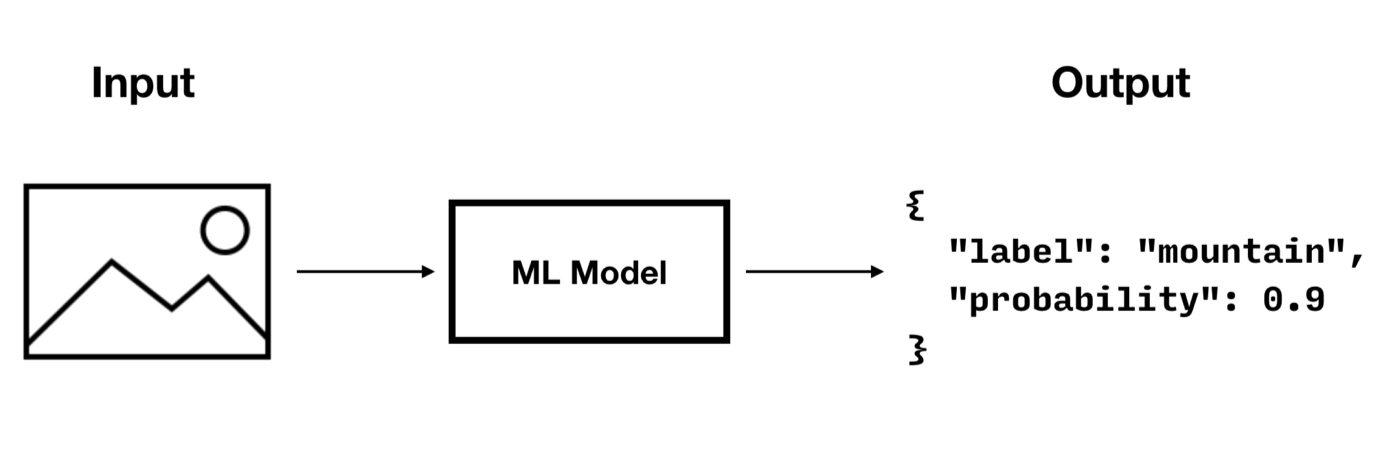# Predictive Queries

+
Couchbase mobile database live query concepts

## Overview

 Enterprise Edition only Predictive Query is an Enterprise Edition feature.

Predictive Query enables Couchbase Lite queries to use machine learning, by providing query functions that can process document data (properties or blobs) via trained ML models.

Let’s consider an image classifier model that takes a picture as input and outputs a label and probability.To run a predictive query with a model as the one shown above, you must implement the following steps.

### Integrate the Model

To integrate a model with Couchbase Lite, you must implement the `PredictiveModel` interface which has only one function called `predict()`.

``````// `myMLModel` is a fake implementation
// this would be the implementation of the ml model you have chosen
class myMLModel {
static func predictImage(data: Data) -> [String : AnyObject] {}
}

class ImageClassifierModel: PredictiveModel {
func predict(input: DictionaryObject) -> DictionaryObject? {
guard let blob = input.blob(forKey: "photo") else {
return nil
}

let imageData = blob.content!
// `myMLModel` is a fake implementation
// this would be the implementation of the ml model you have chosen
let modelOutput = myMLModel.predictImage(data: imageData)

let output = MutableDictionaryObject(data: modelOutput)
return output (1)
}
}``````
 1 The `predict(input) -> output` method provides the input and expects the result of using the machine learning model. The input and output of the predictive model is a `DictionaryObject`. Therefore, the supported data type will be constrained by the data type that the `DictionaryObject` supports.

### Register the Model

To register the model you must create a new instance and pass it to the `Database.prediction.registerModel` static method.

``````let model = ImageClassifierModel()
Database.prediction.registerModel(model, withName: "ImageClassifier")``````

### Create an Index

Creating an index for a predictive query is highly recommended. By computing the predictions during writes and building a prediction index, you can significantly improve the speed of prediction queries (which would otherwise have to be computed during reads).

There are two types of indexes for predictive queries:

#### Value Index

The code below creates a value index from the "label" value of the prediction result. When documents are added or updated, the index will call the prediction function to update the city value in the index.

``````let input = Expression.dictionary(["photo": Expression.property("photo")])
let prediction = PredictiveModel.predict(model: "ImageClassifier", input: input)

let index = IndexBuilder.valueIndex(items: ValueIndexItem.expression(prediction.property("label")))
try database.createIndex(index, withName: "value-index-image-classifier")``````

#### Predictive Index

Predictive Index is a new index type used for predictive query. The Predictive Index is different from the value index in that the Predictive Index caches the predictive result and creates the value index from the cached predictive result when the predictive results values are specified.

The code below creates a predictive index from the "label" value of the prediction result.

``````let input = Expression.dictionary(["photo": Expression.property("photo")])

let index = IndexBuilder.predictiveIndex(model: "ImageClassifier", input: input)
try database.createIndex(index, withName: "predictive-index-image-classifier")``````

### Run a Prediction Query

The code below creates a query that calls the prediction function to return the "label" value for the first 10 results in the database.

``````let input = Expression.dictionary(["photo": Expression.property("photo")])
let prediction = PredictiveModel.predict(model: "ImageClassifier", input: input) (1)

let query = QueryBuilder
.select(SelectResult.all())
.from(DataSource.database(database))
.where(
prediction.property("label").equalTo(Expression.string("car"))
.and(
prediction.property("probablity")
.greaterThanOrEqualTo(Expression.double(0.8))
)
)

// Run the query.
do {
let result = try query.execute()
print("Number of rows :: \(result.allResults().count)")
} catch {
fatalError("Error running the query")
}``````
1 The `PredictiveModel.predict()` method returns a constructed Prediction Function object which can be used further to specify a property value extracted from the output dictionary of the `PredictiveModel.predict()` function.
 The null value returned by the prediction method will be interpreted as MISSING value in queries.

### Unregister the Model

To unregister the model you must call the `Database.prediction.unregisterModel` static method.

``Database.prediction.unregisterModel(withName: "ImageClassifier")``

## Integrate a Model with CoreMLPredictiveModel (iOS only)

`CoreMLPredictiveModel` is a Core ML based implementation of the `PredictiveModel` protocol that facilitates the integration of Core ML models with Couchbase Lite.

The following example describes how to load a Core ML model using `CoreMLPredictiveModel`. All other steps (register, indexing, query, unregister) are the same as with a model that is integrated using your own `PredictiveModel` implementation.

``````// Load MLModel from `ImageClassifier.mlmodel`
let modelURL = Bundle.main.url(forResource: "ImageClassifier", withExtension: "mlmodel")!
let compiledModelURL = try MLModel.compileModel(at: modelURL)
let model = try MLModel(contentsOf: compiledModelURL)
let predictiveModel = CoreMLPredictiveModel(mlModel: model)

// Register model
Database.prediction.registerModel(predictiveModel, withName: "ImageClassifier")``````